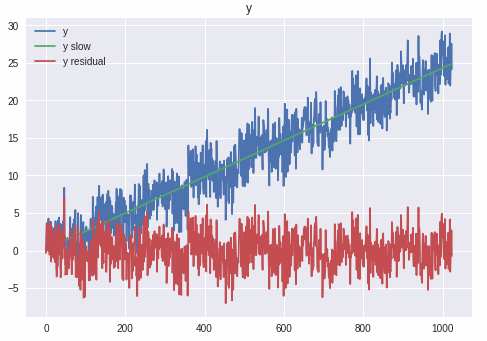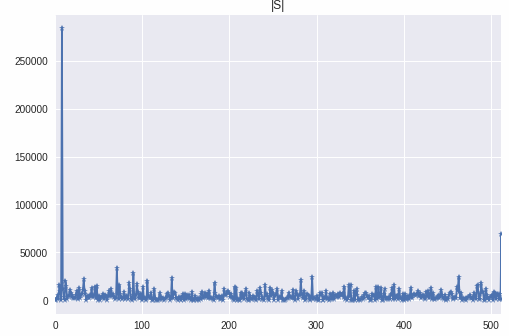#### 有什么方法可以找到时间序列的周期？

统计/机器学习 时间序列 开放问题    浏览次数：3541        分享

0zhaijing   2018-12-05 12:10

##### 3个回答
4

import numpy as np
from numpy.fft import fft,ifft
import matplotlib.pyplot as plt
from sklearn import linear_model
N=1024 # days
m=8 # cycles
# np.random.seed(0)
x = np.linspace(0,m*2*np.pi, N) # N days= m cycles, N/m days/cycle
y0=np.sin(x+1.)
SNR=1./10
noise_std=y0.std()*np.sqrt(1/SNR)
y=y0+np.random.randn(N)*noise_std+x*.5
# y=y-y.mean()
# y=y/y.std()

regr = linear_model.LinearRegression()
regr.fit(x.reshape([-1,1]), y.reshape([-1,1]))
y_slow = regr.predict(x.reshape([-1,1])).flatten()
# y_slow=0
y_residual=y-y_slow

plt.plot(y,label='y')
plt.plot(y_slow,label='y slow')
plt.plot(y_residual,label='y residual')
plt.title('y')
plt.legend()
plt.show()

# FFT to calculate auto-correlation
y_fft=fft(y_residual)
S=y_fft*y_fft
y_corr=ifft(S)
plt.plot(np.absolute(S),'-*')
plt.xlim([0,N/2])
plt.title('|S|')
plt.show()

cycles=np.argmax(np.absolute(S)[:N/2])
print ('# of cycles=%d'%cycles)
print ('%d days/cycle'%(N*1./cycles))
print ('frequency =%.5f'%(cycles*1./N))
# temp=S*0
# temp[max_index]=1
# y_corr=ifft(temp)
# plt.plot(y_corr)
# plt.show()# of cycles=8
128 days/cycle
frequency =0.00781

SofaSofa数据科学社区DS面试题库 DS面经Zealing   2019-03-13 03:20

Thank you! - shilongcn   2019-03-14 14:05
3

SofaSofa数据科学社区DS面试题库 DS面经LiShanfei   2019-02-17 11:37

2

SofaSofa数据科学社区DS面试题库 DS面经gudong321   2019-03-12 17:17相关主题